# Average Rate of Change: Definition, Formula & Examples

Lesson Transcript
Instructor: Ellen Manchester
Finding the average rate of change is similar to finding the slope of a line. Study the definition of average rate of change, its formula, and examples of this concept. Updated: 10/22/2021

## Average Rate of Change = Slope

As we go through life, things tend to change. We are never stuck in one place. Whether it is how much we grow in one year, how much money our business makes each year, or how fast we drive on average. For all of these instances, we would find the average rate of change. The average rate of change is finding how much something changes over time. This is very much like finding the slope of a line.

If you recall, the slope of a line is found by finding the change in y divided by the change in x.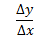This can also be written as the slope formula: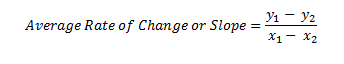The average rate of change and the slope of a line are the same thing. Thinking logically through this formula, we are finding the difference in y divided by the difference in x.

For instance, suppose you have a trip planned. You know you will be traveling through many different areas where the speed limit changes. You will be going 70 mph on one section, then 35 mph on another section. We can find the average speed over the course of the trip by using the slope formula.

Let's take a look at this graph. Time is represented on the horizontal axis, and the speed limit represents the vertical axis. Notice, at the first hour, our speed limit was 30 mph. Here are all the times and speeds represented on this graph.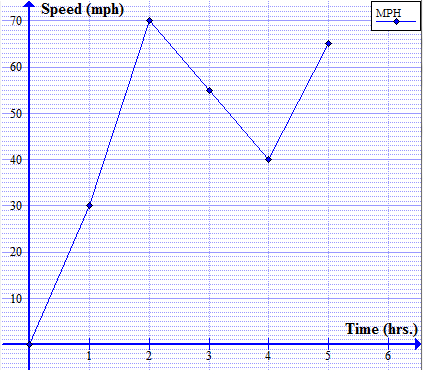• Hour 1: 30 mph
• Hour 2: 70 mph
• Hour 3: 55 mph
• Hour 4: 40 mph
• Hour 5: 65 mph

We can represent these values as our ordered pairs, (x,y): (1,30), (2,70), (3,55), (4,40) and (5,65).

At any two points we can find out the average rate just by finding the slope of the line. Let's find the slope of the line between points at hour 1 and hour 4: (1,30) and (4,40). Using the slope formula, we plug in the values from our ordered pair and solve.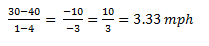This means over the course of three hours our speed changed an average of 3.33 miles every hour. Notice the red line shows the slope or average rate of change as gradual, hence only 3.33 miles per hour.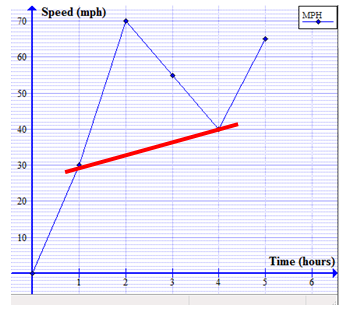Now let's find the average from hour 1 to hour 2: (1,30) and (2,70):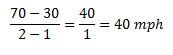Between these two points, our speed changed 40 miles per hour in one hour. The fact that our slope is positive indicates the speed went up or increased. Notice the line goes 'uphill' on this section, which is a positive slope. Also the red line is fairly steep, which indicates a higher average rate increase.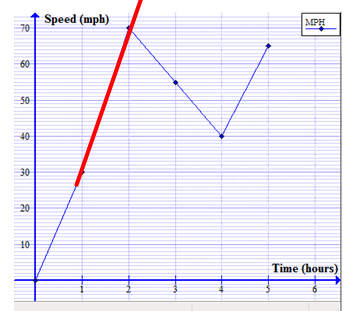Let's look at the rate change from hour 2 to hour 4: (2,70) and (4,40):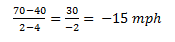This indicates the speed went down or decreased 15 miles per hour because of the negative sign. Notice the slope on the graph is going 'downhill' on this section, which is a decreasing slope and our mph is negative. The red line is showing a negative or decreasing rate.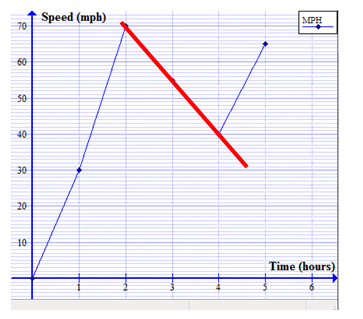As we see here, slope is another version of finding the average rate of change. Average rate of change is finding the difference between the dependent variable (y-term) divided by the difference in the independent variable (x-term). Slope and average rate of change is exactly the same thing. Be sure to keep track of the units in both the numerator and denominator.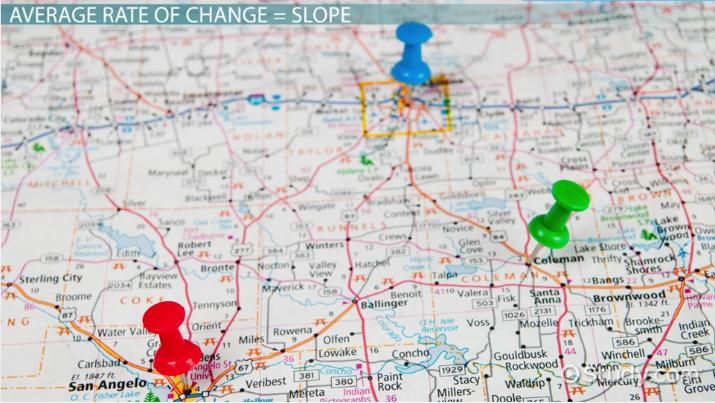An error occurred trying to load this video.

Try refreshing the page, or contact customer support.

Coming up next: Exponent: Definition & Properties

### You're on a roll. Keep up the good work!

Replay
Your next lesson will play in 10 seconds
• 0:01 Average Rate of Change = Slope
• 3:50 More Examples
• 6:06 Lesson Summary
Save Save

Want to watch this again later?

Timeline
Autoplay
Autoplay
Speed Speed

To unlock this lesson you must be a Study.com Member.

## Practice:Average Rate of Change: Definition, Formula & Examples Quiz

1/5 completed

### If the average rate starts out decreasing and continues to decrease, what does the line look like?

Create Your Account To Take This Quiz

As a member, you'll also get unlimited access to over 84,000 lessons in math, English, science, history, and more. Plus, get practice tests, quizzes, and personalized coaching to help you succeed.

It only takes a few minutes to setup and you can cancel any time.

### Register to view this lesson

Are you a student or a teacher?